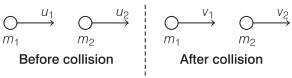# Elastic and Inelastic Collisions in One Dimension | Physics – Work, Energy and Power

One Dimensional or Head-on Collision Definition Physics:
If the initial and final velocities of colliding bodies lie along the same line, then the collision is called one dimensional or head-on collision.

We are giving a detailed and clear sheet on all Physics Notes that are very useful to understand the Basic Physics Concepts.

## Elastic and Inelastic Collisions in One Dimension | Physics – Work, Energy and Power

Perfectly Elastic Collision in One Dimension
Applying Newton’s experimental law, we have
v2 – v1 = u1 – u2Velocities after collision

v1 = $$\frac{\left(m_{1}-m_{2}\right) u_{1}+2 m_{2} u_{2}}{\left(m_{1}+m_{2}\right)}$$

and

v2 = $$\frac{\left(m_{2}-m_{1}\right) u_{2}+2 m_{1} u_{1}}{\left(m_{1}+m_{2}\right)}$$

Special Cases of Elastic Collision in One Dimension:
1. When masses of two colliding bodies are equal, then after the collision, the bodies exchange their velocities.

v1, = u2 and v2 = u1

2. If second body of same mass (m1 = m2) is at rest, then after collision first body comes to rest and second body starts moving with the initial velocity of first body.

v1 = 0 and v2 = u1

3. If a light body of mass m1 collides with a very heavy body of mass m2 at rest, then after collision

v1 = -u1 and v2=0

It means light body will rebound with its own velocity and heavy body will continue to be at rest.

4. If a very heavy body of mass m1 collides with a light body of mass m2(m1 > > m2) at rest, then after collision

v1 = u1 and v2 = 2u1

In Inelastic Collision in One Dimensional
Loss of kinetic energy
ΔK = $$\frac{m_{1} m_{2}}{2\left(m_{1}+m_{2}\right)}\left(u_{1}-u_{2}\right)^{2}\left(1-e^{2}\right)$$

In Perfectly Inelastic One Dimensional Collision
Velocity of separation after collision = 0.

Loss of kinetic energy = $$\frac{m_{1} m_{2}\left(u_{1}-u_{2}\right)^{2}}{2\left(m_{1}+m_{2}\right)}$$If a body is dropped from a height h0 and it strikes the ground with velocity v0 and after inelastic collision it rebounds with velocity v1, and rises to a height h1, thenIf after n collisions with the ground, the body rebounds with a velocity vn and rises to a height hn, then

$$e^{n}=\frac{v_{n}}{v_{0}}=\sqrt{\frac{h_{n}}{h_{0}}}$$

Height covered by the body after rath rebound, hn = e2nh0

Work, Energy and Power:
Work, energy and power are the three quantities which are inter-related to each other. The rate of doing work is called power. An equal amount of energy is consumed to do a work. So, basically the power is the rate at which energy is consumed to complete a work.